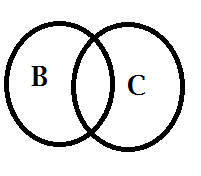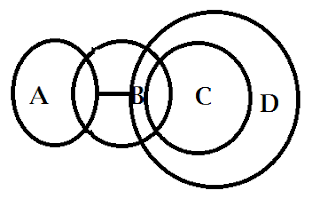New Students Offer - Use Code HELLO

# Tricks to Solve Reverse Syllogism

Syllogism is a game played with circles logically. Usually statements will be given and we have to find the appropriate conclusion which follows the statements. In Reverse Syllogism, as the name suggest, Conclusions will be given and we have to find the appropriate statements which satisfies the conclusions.

It may seems like a lengthy process, But if you are strong in basic concepts of syllogism along with some elimination tricks, you can master this topic.
Let us brush up some basic concepts of syllogism with some examples.

### Example.1

Statement: All A are B#### Possible Conclusions:

1. Some A are B
2. Some B are A
3. Some B are not A

### Example. 2

Statement: Some B is C#### Possible Conclusions:

1. Some C is B
2. Some B is not C
3. Some C is not B
4. All B are C is Possible/ All B may be C
5. All C are B is Possible

Note: Some B are C is Possible cannot be a conclusion
May be/ Can be means Possibility
At least some means Some
Very few means Some

### Example. 3

Statement: No C is D#### Possible Conclusions:

1. No D is C
2. Some C are not D
3. Some D are not C
4. All C can never be D
5. All D can never be C
Now let us combine all the statements of the above three examples.

### Example. 4

Statement:
All A are B
Some B is C
No C is D#### Possible Conclusions:

1. No D is C
2. Some C are not D
3. Some D are not C
4. Some A are not D
5. Some B are not D
6. All A are C is Possible
7. No A is D is Possible
8. No B is D is Possible
9. Some A are D is Possible
10. Some B are D is Possible
11. All B can never be D
12. Some A are D (or) No A is D
13. Some B are D (or) No B is D

## Now Let us discuss the elimination tricks to solve Reverse Syllogism with some examples:

### Example.1

Conclusions:
I. All A can never be B
II. Any A which is a D is also C

Statements:
A. Some A is B. All B is C. Some C is D
B. Some A are D. All A are C. No B is D
C. No A is C. All C are B. Some C is D
D. All A are C. Some C are D. No D is B
E. None of these

#### Explanation:

• First let us take a quick scan on the statements
• Take Option A and compare it with conclusion I,  All A can never be B. Some A is B. All B is C. Some C is D (Option A is eliminated)
• Take Option C and compare it with conclusion II  Any A which is a D is also C No A is C. All C are B. Some C is D (Option C is eliminated)
• Now we have Option B and D
• Take Option B and compare with both the conclusions.
Some A are D. All A are C. No B is D• From the Shaded region it is clear that All A can never be B and Any A which is D is also C. (Both conclusions Follows, so Option B is the answer)
• In Exam You can stop once you find the answer.
• But, Now let us take Option D also and check.

All A are C. Some C are D. No D is B• Here All A can never be B may or may not be true
• Therefore, option D doesn’t follow.

### Example. 2

Conclusions:
I. Atleast Some A may be C.
II. All C being B is a Possibility.

Statements:
A. Some A are B. Some B are C. All C are D. No A is D.
B. All A are B. No C is B. Some A is D
C. No C is A .All A are D. Some D is B
D. All A Are B. Some A are D. No D is C
E. None of these

#### Explanation:

• First let us take a quick scan on the statements
• Take Option B and compare it with conclusion II,  All C being B is a Possibility.  All A are B. No C is B. Some A is D (Option B is Eliminated)
• Take Option C and Compare it with Conclusion I Atleast Some A may be C. No C is A. All A are D. Some D is B (Option C is eliminated)
• Now we have Option A and D
• Take Option A and Compare with both the conclusions
Some A are B. Some B are C. All C are D. No A is D.• Here conclusion I don’t follow
• Now Let us take Option D and Compare with both the conclusions
All A Are B. Some A are D .No D is C• Both the Conclusions Follow. To understand the concept more easily, above diagram can be redrawn as.